# Bar Graph Worksheets

Bar graph worksheets contain counting objects, graphing by coloring, comparing tally marks, creating graph, reading bar graph, double bar graph, drawing bar graph to represent the data, making your own survey and more. Each worksheet contains unique theme to clearly understand the usage and necessity of bar graph in real-life.

Counting and Coloring Graph

Color the graph to show the count of each picture. Answer the questions based on the bar graph.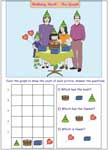Pond Scenery

Tally Marks and Bar Graph

Counting of each object is represented by tally marks. Draw and color the bar graph to show the count.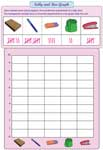Charity Clothes

Counting and Creating Graph

You have a collection of objects in each worksheet. Count the number of objects in each kind and draw a bar graph to represent the counting.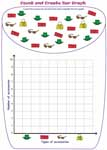Each worksheet has 5 questions based on reading bar graph. Scale increments by 1s. These worksheets are suitable for grade one kids.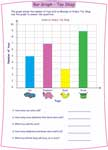Read the bar graph and answer the questions. Scale increments by 2s and 5s. There are 5 bars in each worksheet.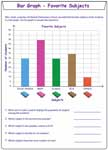Reading bar graph goes little hard this time. The increments are two-digit and three-digit numbers. Each worksheet has six bars and five word problems based on bars.Double Bar Graph

There are five set of double bar graphs in each worksheet. It helps to improve your decision making skills by comparing and analyzing the product or information.Gone Fishing

Drawing Bar Graph: Easy Level

Draw a bar graph to represent the data. Use the information to answer the questions.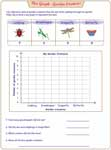Drawing Bar Graph: Moderate Level

Bar graph worksheets in this page contain scale increments by 4s and 8s. There are four questions in each worksheet.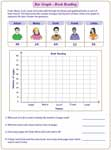Donations

Drawing Bar Graph: Difficult Level

At this level, scale increments are huge and the quantities are in 2-digits, hundreds and thousands.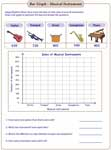Take a survey among your friends and family members. Record the results in this chart and draw a bar graph to represent your data.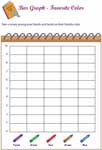Title, Labeling Axis, Scaling and Graphing

Using the given information, write a title of the bar graph, label x and y axis, make appropriate scale, and draw a graph to represent the data.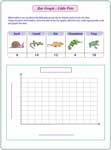Favorite Animals

Related Worksheets